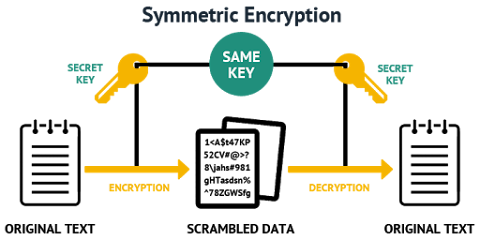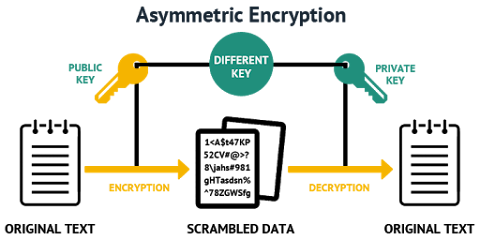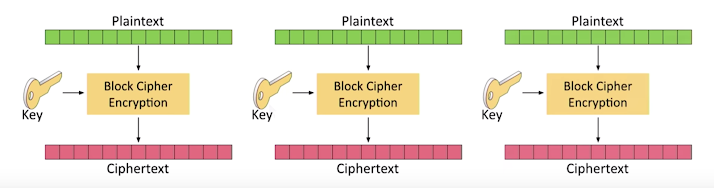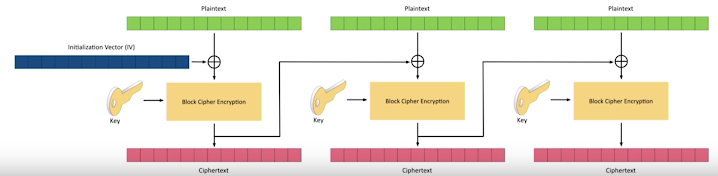Cryptography

## Encryption Algorithms

Encryption systems are often grouped into families. Common families include symmetric systems (e.g. AES) and asymmetric systems (e.g. RSA); They may alternatively be grouped according to the central algorithm used (e.g. elliptic curve cryptography).

Table 1. Major Difference
- Symmetric Asymmetric

Keys

Single Key(Secret Key)

Two Keys (Public & Private)

KeyLength

-

1024, 2048, 4096

Algorithms

RSA, ECC

Performance

Fast in its execution

Slower in execution as a result of more complex algorithms which come with a high computation burden.

Purpose

Bulk data encryption(text file encryption)

Asymmetric encryption used mostly used for securely exchange secret keys(of symmetric encryption)

## Symmetric Algorithm (AES)

AES is a Symmetric encryption algorithm also called SecretKey Encryption.

It employs the same secret key for both encryption and decryption. Large amounts of data can be encrypted using a symmetric encryption algorithm.### Initialization Vector(IV) (Nonce)

Initialization Vector(IV) is generated before encryption initialization, which is a number of bytes. Generally, it should be random or pseudo-random. This setting does not allow an attacker to get relations between encrypted messages segments, making a hack more complicate therefore.

 Some cryptographic primitives require the IV only to be non-repeating, and the required randomness is derived internally. In this case, the IV is commonly called a `NONCE(number used once)`.

## Asymmetric Algorithm (RSA)

`RSA Stands for Rivest, Shamir, and Adelman.` It also called Public Key Cryptography.

An asymmetric encryption algorithm, based on using public and private keys. A message is encrypted using a public key and can be decrypted only with a private key.Unlike symmetric encryption, asymmetric encryption is a heavy operation and requires significant computer power.

 There is limit on message length which can be encrypted using an asymmetric algorithm depending on a key length.
 Typically, this(asymmetric) algorithm is used to obtain a key of the symmetric encryption algorithms by which an encryption takes place after, and for digital certificates signing.

RSA key length is 1024, 2048, 4096 bits.

RSA uses certain encryption schemes that applies algorithms of adding data which means nothing to encrypted information, aimed at improving process reliability:

• RSA-OAEP

• RSA-PKCS1-v1_5

In cryptography `padding` refers to a number of distinct practices.

The primary use of padding with classical ciphers is to prevent the cryptanalyst from using that predictability to find known plaintext that aids in breaking the encryption.

 Random length padding also prevents an attacker from knowing the exact length of the plaintext message.
 It should be noted that RSA-PKCS1-v1_5 scheme is considered to be insufficiently reliable, you better use RSA-OAEP.

## Symmetric Algorithms Types

### DES (Data Encryption Standard)

The Data Encryption Standard (DES) is an outdated symmetric-key method of data encryption.

The Data Encryption Standard is a block cipher, meaning a cryptographic key and algorithm are applied to a block of data simultaneously rather than one bit at a time.

To encrypt a plaintext message, DES groups it into 64-bit blocks. Each block is enciphered using the secret key into a 64-bit ciphertext by means of permutation and substitution.

The process involves 16 rounds and can run in four different modes, encrypting blocks individually or making each cipher block dependent on all the previous blocks.

Decryption is simply the inverse of encryption, following the same steps but reversing the order in which the keys are applied.

For any cipher, the most basic method of attack is brute force, which involves trying each key until you find the right one. The length of the key determines the number of possible keys — and hence the feasibility — of this type of attack. DES uses a 64-bit key,

but eight of those bits are used for parity checks, effectively limiting the key to `56-bits`.

Hence, it would take a maximum of 2^56, or 72,057,594,037,927,936, attempts to find the correct key.

des_key_generation.java
``````   public static String generateSecretKey() throws NoSuchAlgorithmException {

KeyGenerator keyGenerator = KeyGenerator.getInstance("DES");
keyGenerator.init(56);// Must be 56 for DES

LOGGER.info(" Algorithm {} and provider {}", keyGenerator.getAlgorithm(), keyGenerator.getProvider().getName());

SecretKey secretKey = keyGenerator.generateKey();

LOGGER.info(" Encoded Secret bytes {} ",secretKey.getEncoded());

LOGGER.info("secretKey {}  and Format {}",secretKey.getAlgorithm(), secretKey.getFormat());
return new BASE64Encoder().encode(secretKey.getEncoded());
}``````

### 3DES

Triple DES (3DES), officially the Triple Data Encryption Algorithm (TDEA or Triple DEA), is a symmetric-key block cipher, which applies the DES cipher algorithm three times to each data block.

### RC4

RC4 is a stream cipher and variable length key algorithm. This algorithm encrypt one byte at a time (or larger units on a time).

RC4 generates a pseudo-random stream of bits (a key-stream). As with any stream cipher, these can be used for encryption by combining it with the plaintext using bit-wise exclusive-or. Decryption is performed the same way (since exclusive-or is a symmetric operation).

In cryptography, the QUAD, cipher is a relatively new stream cipher, which was designed with provable security arguments in mind.

### AES

AES is symmetric encryption which works with single key called `Secret Key.`

#### Generate Secret Key

GenerateKey.java
``````public static String generateSecretKey() throws NoSuchAlgorithmException {

KeyGenerator keyGenerator = KeyGenerator.getInstance("AES");
keyGenerator.init(128);// Must be 56 for DES

LOGGER.info(" Algorithm {} and provider {}", keyGenerator.getAlgorithm(), keyGenerator.getProvider().getName());

SecretKey secretKey = keyGenerator.generateKey();

LOGGER.info(" Encoded Secret bytes {} ", secretKey.getEncoded());

LOGGER.info("secretKey {}  and Format {}", secretKey.getAlgorithm(), secretKey.getFormat());
return new BASE64Encoder().encode(secretKey.getEncoded());
}``````

#### Encryption with AES

Encryption.java
`````` public static String encrypt(String plainText, String secretKeyString) throws Exception {

byte[] plainTextBytes = plainText.getBytes();

byte[] secretKeyBytes = new BASE64Decoder().decodeBuffer(secretKeyString);

SecretKey secretKey = new SecretKeySpec(secretKeyBytes, "AES");

cipher.init(Cipher.ENCRYPT_MODE, secretKey);

return new BASE64Encoder().encode(cipher.doFinal(plainTextBytes));

}``````

#### Decryption with AES

Decrypt.java
``````    public static String decrypt(String encryptedString, String secretKeyString) throws Exception{

byte[] secretKeyBytes=new BASE64Decoder().decodeBuffer(secretKeyString);
SecretKey secretKey = new SecretKeySpec(secretKeyBytes, "AES");
cipher.init(Cipher.DECRYPT_MODE,secretKey);

return new String(cipher.doFinal(new BASE64Decoder().decodeBuffer(encryptedString)));

}``````

## Block Cipher Modes

also called `Encryption Modes`

One way of encryption is to encrypt one fixed-length group of bits at a time called A Block. This can be achieved with many different ways called mode of operation.

Mode of operation defines

• Defines the method of encryption

• May provide a method of authentication

The block size is fixed size

• Not all data matches the block size perfectly

• Split your plaintext into smaller blocks

• Some modes require padding before encryption

An Important parameter for AES is the `encryption mode`. It defines, how the sequence of open data blocks converted into encrypted ones.

Mode of operation describes how to repeatedly apply a cipher’s single-block operation to securely transform amounts of data larger than a block.

For AES, there are such modes:

• ECB

• CBC

• PCBC

• CFB

• OFB

• CTR

### ECB

`Electronic CodBook`

It is the simplest mode of encryption. Each plaintext block is encrypted separately.

Similarly, each ciphertext block is decrypted separately.

Thus, it is possible to encrypt and decrypt by using many threads simultaneously.

However, in this mode the created ciphertext is not blurred.A typical example of weakness of encryption using ECB mode is encoding a bitmap image (for example a .bmp file). Even a strong encryption algorithm used in ECB mode cannot blur efficiently the plaintext.

A message that is encrypted using the ECB mode should be extended until a size that is equal to an integer multiple of the single block length.

A popular method of aligning the length of the last block is about appending an additional bit equal to 1 and then filling the rest of the block with bits equal to 0.

It allows to determine precisely the end of the original message.

 ECB all plaintext blocks must be equal size

Apart from revealing the hints regarding the content of plaintext, the ciphers that are used in ECB mode are also more vulnerable to replay attacks.

### CBC

`Cipher Block Chaining`

It is popular mode of operation that is relatively easy to implement.

How does it works

Each plaintext block is XORed with the previous cipher text block, which adds additional randomization.

`Initialization Vector` is required for initial step of encryption process.Encryption in CBC mode can only be performed by using one thread.

Despite this disadvantage, this is a very popular way of using block ciphers.

CBC mode is used in many applications.

If one bit of a plaintext message is damaged (for example because of some earlier transmission error),

all subsequent ciphertext blocks will be damaged and it will be never possible to decrypt the ciphertext received from this plaintext.

As opposed to that, if one ciphertext bit is damaged, only two received plaintext blocks will be damaged. It might be possible to recover the data.

### PCBC

`Propagating or Plaintext Cipher-Block Chaining Mode`

The PCBC mode is similar to the previously described CBC mode. It also mixes bits from the previous and current plaintext blocks, before encrypting them. In contrast to the CBC mode, if one ciphertext bit is damaged, the next plaintext block and all subsequent blocks will be damaged and unable to be decrypted correctly.

In the PCBC mode both encryption and decryption can be performed using only one thread at a time.

### CFB

`Cipher Feedback Mode`

The CFB mode is similar to the CBC mode described above.

The main difference is that one should encrypt ciphertext data from the previous round (so not the plaintext block)

and then add the output to the plaintext bits. It does not affect the cipher security but it results in the fact that the same encryption

algorithm (as was used for encrypting plaintext data) should be used during the decryption process.

### OFB

`Output Feedback Mode`

Algorithms that work in the OFB mode create keystream bits that are used for encryption subsequent data blocks.

In this regard, the way of working of the block cipher becomes similar to the way of working of a typical stream cipher.### CTR

`Counter Mode`

Using the CTR mode makes block cipher way of working similar to a stream cipher. As in the OFB mode, keystream bits are created regardless of content of encrypting data blocks. In this mode, subsequent values of an increasing counter are added to a nonce value (the nonce means a number that is unique: number used once) and the results are encrypted as usual. The nonce plays the same role as initialization vectors in the previous modes.It is one of the most popular block ciphers modes of operation. Both encryption and decryption can be performed using many threads at the same time.

If one bit of a plaintext or ciphertext message is damaged, only one corresponding output bit is damaged as well.

Thus, it is possible to use various correction algorithms to restore the previous value of damaged parts of received messages.

The CTR mode is also known as the SIC mode (Segment Integer Counter).

### Reference

Padding standards are mechanisms for appending some predefined values to messages.

They are used with algorithms which deal with blocks of data.

Typical examples of such operations are block symmetric ciphers and MAC algorithms.

These algorithms work on the whole data blocks. Therefore, if a message length is not a multiple of the block size,

a standard for adding some number of bytes to the end of the message is required.

The information which padding standard has been used, must be provided to the receiver.

This allows them to determine (after decrypting the ciphertext) where the original message ends, and the unimportant pad bytes starts.

All the padding standards defined below work in a similar way. They describe which values should be appended to the message, to fill up the last block.

Using padding is a convenient way of making sure that encrypted data is of the correct size.

The only drawback is the fact that even if the original message contains the correct number of bytes (a multiple of the block size),

some padding must be added to fulfil the process and make sure that the receiver would be able to understand the message.

Usually, a new dummy block must be added which will contain only the padding bytes.

There are a few padding types described below. The first two paddings are based on bits, whereas the others are based on bytes.

A single 1 bit is appended to the data. Then, all other bits of the padding (if any are required) are zeros.

`1 0 1 0 0 0 0 1 1 0    1 0 0 0 0 0`

This padding scheme is defined in ISO/IEC 9797-1 documentation.

### TBC (Trailing Bit Complement) Padding

If the data ends in a 0 bit, all the padding bits will be ones. If the data ends in a 1 bit, all the padding bits will be zeros.

```1 0 1 0 0 0 0 1 1 0    1 1 1 1 1 1
1 0 1 0 0 0 0 1 1 1    0 0 0 0 0 0```

The value of each pad byte is the total number of bytes that are added. Of course, the total number of pad bytes depends on the block size.

For example, if the message is 3 bytes shorter than an integer multiple of the block size, then 3 pad bytes should be added, each of them of value 3. If 5 bytes should be added, then each of them should be 5.

```0x10 0x11 0x36 0x67 0x38 0xBC 0x03 0x21 0xEF  0x03 0x03 0x03
0x10 0x11 0x36 0x67 0x38 0xBC  0x06 0x06 0x06 0x06 0x06 0x06```

The first byte of the padding is 0x80. All other bytes of the padding are zeros. Such construction allows to create paddings of any size.

`0x10 0x11 0x36 0x67 0x38 0xBC 0x03 0x21 0xEF  0x80 0x00 0x00`

The padding mechanism is defined in ISO/IEC 7816-4 documentation.

The last byte of the padding (thus, the last byte of the block) is the number of pad bytes. All other bytes of the padding are some random data.

`0x10 0x11 0x36 0x67 0x38 0xBC 0x03 0x21 0xEF  0x23 0x86 0x03`

The padding mechanism is defined in ISO 10126-2 documentation.

The last byte of the padding (thus, the last byte of the block) is the number of pad bytes. All other bytes of the padding are zeros.

`0x10 0x11 0x36 0x67 0x38 0xBC 0x03 0x21 0xEF  0x00 0x00 0x03`

The padding mechanism is defined in the ANSI X9.23 standard.

```0x10 0x11 0x36 0x67 0x38 0xBC 0x03 0x21 0xEF  0x00 0x00 0x00